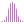#ExpRegPoint (PQL)

Calculates the exponential regression of a set, and returns the value of the y-intercept in the regression line for a particular value of x.

#### Syntax

ExpRegPoint( <Numeric> , <List> , <Numeric> , OPTIONAL <Numeric> )

* Click on the function's arguments above for more details on the input values.